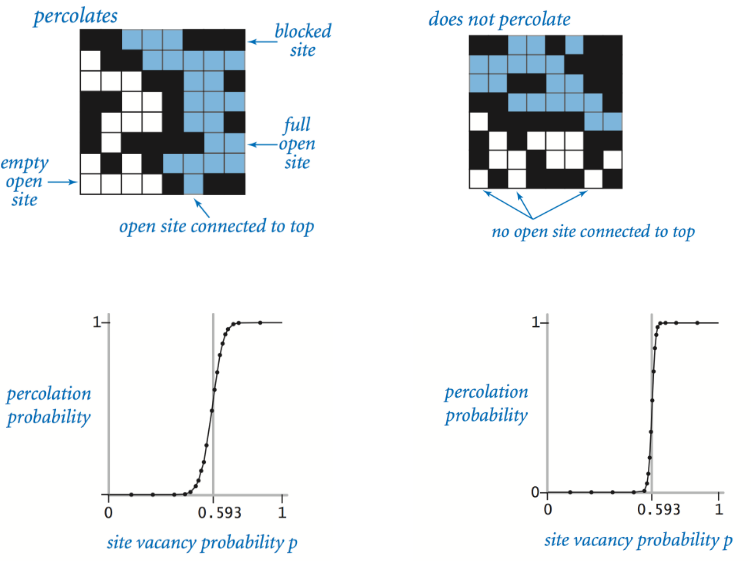This problem appeared as an exercise in the Coursera Course Algorithm-I (By Prof. ROBERT SEDGEWICK, Princeton) as an application of the Union-find.

### Percolation

(as defined in *http://coursera.cs.princeton.edu/algs4/assignments/percolation.html*)

Given a composite systems comprised of randomly distributed insulating and metallic materials: what fraction of the materials need to be metallic so that the composite system is an electrical conductor? Given a porous landscape with water on the surface (or oil below), under what conditions will the water be able to drain through to the bottom (or the oil to gush through to the surface)? Scientists have defined an abstract process known as percolation to model such situations.

### The model

A percolation system is modeled using an n-by-n grid of sites. Each site is either open or blocked. A full site is an open site that can be connected to an open site in the top row via a chain of neighboring (left, right, up, down) open sites. The system is said to percolate if there is a full site in the bottom row. In other words, a system percolates if we fill all open sites connected to the top row and that process fills some open site on the bottom row. (For the insulating/metallic materials example, the open sites correspond to metallic materials, so that a system that percolates has a metallic path from top to bottom, with full sites conducting. For the porous substance example, the open sites correspond to empty space through which water might flow, so that a system that percolates lets water fill open sites, flowing from top to bottom.)

### The problem

In a famous scientific problem, researchers are interested in the following question: if sites are independently set to be open with probability p (and therefore blocked with probability 1 - p), what is the probability that the system percolates? When p equals 0, the system does not percolate; when p equals 1, the system percolates. The plots below show the site vacancy probability p versus the percolation probability for 20-by-20 random grid (left) and 100-by-100 random grid (right).When n is sufficiently large, there is a threshold value p* such that when p < p* a random n-by-n grid almost never percolates, and when p > p*, a random n-by-n grid almost always percolates. No mathematical solution for determining the percolation threshold p* has yet been derived. We shall estimate p* with Monte Carlo simulation.

### Monte Carlo simulation

To estimate the percolation threshold, let’s consider the following computational experiment:

1. Initialize all sites to be blocked.
2. Repeat the following until the system percolates:
3. Choose a site uniformly at random among all blocked sites.
4. Open the site.
5. The fraction of sites that are opened when the system percolates provides an estimate of the percolation threshold.
• By repeating this computation experiment T times and averaging the results, we obtain a more accurate estimate of the percolation threshold. Let $$x_t$$ be the fraction of open sites in computational experiment t. The sample mean $$\bar{x}$$ provides an estimate of the percolation threshold; the sample standard deviation s measures the sharpness of the threshold.

• Assuming T is sufficiently large (say, at least 30, by CLT), we can construct a 95% confidence interval for the percolation threshold as follows.